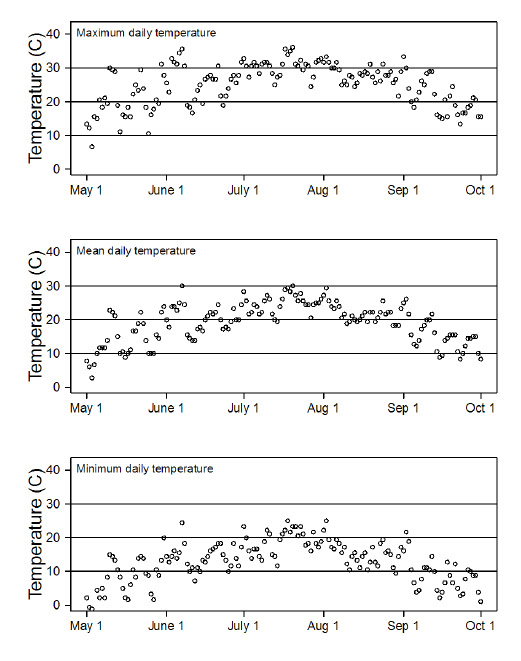Ecological Archives E095-131-A1

Brandon T. Barton, Anthony R. Ives. 2014. Direct and indirect effects of warming on aphids, their predators, and ant mutualists. Ecology 95:1479–1484. http://dx.doi.org/10.1890/13-1977.1

Appendix A. Statistical results and daily temperature data from the field site.

Table A1. Repeated-measures linear mixed model (LMM) results showing the response of ant abundance (square-root transformed) to ant species (A), predator presence (P), and warming (W) through time (Eq. 2). Interactions not presented were not significant. The autocorrelation among observations of the same replicate r = 0.075, and the variance σ² depended on the mean m according to σ² = exp(0.13 m). N = 192 in 48 replicates (plants).

 Coefficient χ² df P A 0.003 1 0.91 time 0.15 3 0.93 A × time 1.8 3 0.54 P × time 2.1 4 0.74 W × time 8.4 4 0.37 A × W × time 30.4 4 <0.0001

Table A2. GLMM results showing whether or not ladybeetle larvae were attacked in <60s by ant species (A) and temperature (W) (Eq. 3). The A × W interaction was not significant. The variance for the group random effect σ² = 0.24. N = 48 in 8 groups (dates).

 Coefficient Estimate SD Z P A 2.62 0.72 3.63 0.00028 W -0.03 0.034 -0.88 0.38

Table A3. LMM results showing the time (square-root transformed) to attack a ladybeetle larva (given an attack occurred) by ant species (A) and temperature (W) (Eq. 4). The A × W interaction was not significant. The variance for the group random effect σ² = 0. N = 25 in 8 groups (dates).

 Coefficient Estimate SD t P A -1.40 0.68 -2.06 0.052 W 0.066 0.028 2.38 0.026

Table A4. LMM results showing the time (square-root transformed) to attack a ladybeetle larva (given an attack occurred) by ant species (A). The variance for the group random effect σ² = 0. N = 25 in 8 groups (dates).

 Coefficient Estimate SD t P A -1.83 0.72 -2.53 0.019

Table A5. LMM results showing the time (square-root transformed) to attack a ladybeetle larva (given an attack occurred) by the winter ant as a function of temperature (W). The variance for the group random effect σ² = 0. N = 19 in 8 groups (dates).

 Coefficient Estimate SD t P W 0.087 0.028 3.10 0.0065Fig. A1. Temperature data from 1 May 2011 to 1 October 2011 at the Dane County Regional Airport, Madison, WI. This weather station is approximately 25 km south of the University of Wisconsin Arlington Agricultural Research Station where the field experiment was conducted. Temperature data was downloaded from WeatherUnderground. (http://www.wunderground.com/history/airport/KMSN/2011/5/1/CustomHistory.html?dayend=1&monthend=10&yearend=2011&req_city=NA&req_state=NA&req_statename=NA)